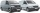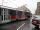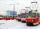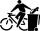Car overtaking

A passenger car travels at a speed of 30 m/s, and before it travels freight truck that drives at a constant speed of 10 m/s. Within 30 meters of the truck, the driver of the car finds out that the truck can not overtake. That's why it starts braking with an acceleration of 5 m/s. Will cars collide? If so, how big are the car speeds at the time of the collision?

Result

v22 =  12.679 m/s

Solution:Leave us a comment of this math problem and its solution (i.e. if it is still somewhat unclear...):Be the first to comment!To solve this verbal math problem are needed these knowledge from mathematics:

Do you want to convert length units?

Next similar math problems:

1. WavelengthCalculate the wavelength of the tone frequency 11 kHz if the sound travels at speeds of 343 m/s.
2. Two vansAt 8:30 a. M. , two vans leave at a parking area. They travel in opposite directions: one van travels at 48 km/h and the other at 62 km/h. At what time will the cars be 550 km apart?
3. GravitationFrom the top of the 80m high tower, the body is thrown horizontally with an initial speed of 15 m/s. At what time and at what distance from the foot of the tower does the body hit the horizontal surface of the Earth? (use g = 10 ms-2)
4. Free fallThe free fall body has gone 10m in the last 0.5s. Find the body speed at the moment of impact.
5. BomberThe aircraft flies at an altitude of 4100 m above the ground at speed 777 km/h. At what horizontal distance from the point B should be release any body from the aircraft body to fall into point B? (g = 9.81 m/s2)
6. G forcesCalculate deceleration of car (as multiple of gravitational acceleration g = 9.81 m/s2) which occurs when a car in a frontal collision slows down uniformly from a speed 111 km/h to 0 km/h in 1.2 meters trajectory.
7. DriverThe driver of the car at a speed of 100 km/h faced the obstacle and began to brake with a slowing of 5 m/s². What is the path to stopping the car when the driver has registered the obstacle with a delay of 0.7 s?
8. TramsTrams have an average speed 23 km/h and run in tact 14 minutes. Pedestrian walking speed is 3.3 km/h. At what intervals trams outrun pedestrian?
9. CollisionThe two bodies, whose initial distance is 240 m, move evenly against each other consistently. The first body has an initial velocity of 4 m/s and an acceleration of 3 m/s2, the second body has an initial speed of 6 m/s and an acceleration of 2 m/s2. Find
10. Aircraft nose downHow long will fall airliner from a height of 10000 m at speed 1,000 km/h?
11. The tramThe tram is moving with acceleration a = 0.3m/s2. How long it will pass the first meter of track? How long does it take 10 meters. What is its speed at the end of the 10 meters track?
12. Cyclist and carA cyclist who runs at a speed of 20km per hour will arrive at the finish in 4.5 hours. How fast does the car have to drive the same route in 2 hours?
13. Constant motionThe car has undergone constant motion 120 km in 1 hour 30 minutes. Determine its average speed. Determine how long car drove in the first 20 minutes and 50 minutes from the start of the move.
14. Athletic competitionIn a 400 meter athletic competition, a participant covers the distance as given below. find the average speed? first 80 meters 10 m/s next 240 meters 7.5 m/s last 80 meters 10 m/s
15. AbyssStone was pushed into the abyss: 2 seconds after we heard hitting the bottom. How deep is the abyss (neglecting air resistance)? (gravitational acceleration g = 9.81 m/s2 and the speed of sound in air v = 343 m/s)
16. Train 2The train slowed down from 90 km/h to 72 km/h in 5 seconds. How long track travel?
17. Cyclist vs carCyclist rode out of the city at 18 km/h. 1 hour 30 minutes behind him started car and caught up with the cyclist in 50 minutes. How fast was driving the car? Where (what kilometer) from the city car overtook a cyclist?